# Using an ATR Rotator to Study Oriented Samples

Majority of single crystals are oriented, while polymers, plastics and liquids are not usually oriented. However, certain manufacturing methods may create preferential orientation of molecules along specific machining directions.

Oriented samples are defined by chemical bonds that are aligned in a specific direction or axis. In general, oriented samples will exhibit different chemical and mechanical properties, both perpendicular and along the axis.

In order to match the properties of oriented samples with the degree of orientation, the samples must be examined both during material development and production phase for quality check purposes.

This article explores how ATR measurements are used for studying orientation. The Seagull variable angle accessory is specifically utilized with its ATR Rotator and wire grid polarizer for fixed 67° measurements of a plastic.

## Theory

The fundamental principle utilized for measuring molecular orientation through spectroscopic techniques depends on the amount of radiation taken in by a sample for different orientations of the electric field of the polarized incident light with regard to the sample. The absorbance thus determined for a specific chemical bond in a specific direction is proportional to the average of the square of the cosine of the angle of the dipoles relating to this chemical bond and the direction.

When the ratio of two absorbances is calculated in two directions, it is possible to get the ratio of the average square cosines for these directions. These are simply geometric data on the sample’s molecular structure. For example, in transmission spectroscopy, it is possible to obtain measurements at standard incidence by either turning the polarization of the incident light and determining the absorbance for varied angles of rotation, or by rotating the sample with the polarizer kept in a fixed position.

In order to measure the degree of orientation, let us take a simple model in which a certain fraction (f) of molecular dipoles is suitably oriented in a specific direction and the reminder (1-f) is oriented in a random direction. It is possible to determine both absorbance A1 and absorbance A2 for the field oriented along that direction and the field perpendicular to that direction, respectively.

The absorbance A1 is the result of the fraction f oriented along this direction in addition to (1-f)/3 of the randomly oriented bonds as shown in Figure 1, while the absorbance A2 occurs mainly due to (1-f)/3 of the randomly oriented bonds, resulting in the ratio of (1+2f)/(1-f). This helps in measuring the fraction f.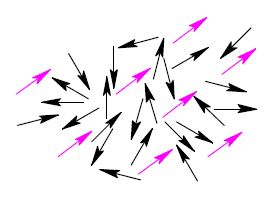Figure 1. Two dimensional illustration of the oriented fraction f of dipoles (magenta) imbedded in a background of randomly oriented dipoles (black).

Both transmission spectroscopy and the above-illustrated model are restricted to optically thin samples. However, ATR is not limited by the thickness of samples. In the latter approach, the absorption occurs from the interaction between the evanescent wave and the sample. Harrick has given the electric fields of the evanescent wave at the interface as follows: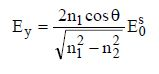(1)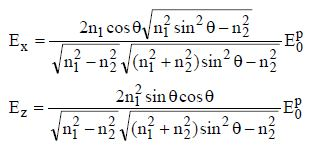(2)

where E0 refers to the electric field of the incident light, θ is the angle of incidence, n1 is the refractive index of the ATR element and n2 is the refractive index of the sample. In case of s-polarized incident light, Ey alone exists while Ex and Ez remain zero. For p-polarized incident light, Ey is zero, whereas both Ex and Ez Ex are nonzero.

Therefore, the absorbance largely relies on the field strength for a specific direction, besides the average cosine squared for that direction and bond. To establish the geometric data of the sample’s molecular structure, it is essential to obtain the ratio of the field strengths squared for the two directions from the ratio of the quantified absorbances to achieve the ratio of cosines squared for the two directions.

It should be noted that the refractive index of the sample n2 remains the same in all directions, but this is often not true for oriented samples. The refractive index of anisotropic samples usually depends on direction, but for poor absorption the dependence of the refractive index on the direction is rather weak and is usually overlooked.

In case of s-polarized light, the electric field of the incident light rotates perpendicular to the plane of incidence. In other words, it is along the y- axis - pointing out of paper in Figure 2.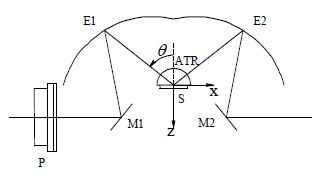Figure 2. Geometry of the experiment.

Therefore, the induced-evanescent wave in the sample exhibits an electric field oscillating in the y-axis direction. This field can only activate those dipoles in the sample containing components along the y-axis, and hence, the dipoles oriented along the x and z directions are not excited. Conversely, if the sample can oscillate around z-axis, turning the sample by 90° interchanges both x and y directions.

The same evanescent wave can activate dipoles originally pointing in the x-direction and now pointing in the y-direction. Thus, x and y directions can be studied by just oscillating the sample. The spectral dependence on sample orientation can be completely studied if the sample can rotate to any angle.

Chemical bond orientation can be inferred from the angular plots of peak height versus angle, referenced to the sample’s external dimensions. To probe perpendicular to the surface of the sample and into the thickness of the sample (z-axis), the electric field should rotate in the z-direction.

With p-polarized incident light, the induced-evanescent wave in the sample exhibits an electric field that oscillates in the xz plane. This electric field oscillates only at critical angle and mainly in the z-direction, but experimental measurements performed at the critical angle create spectra that are quite distorted.

In reality, to make spectra free of distortion, the angle of incidence must be several degrees above the critical angle. Hence, x and z directions are always studied together. However, when the angle of incidence rises, the relative strength of the field in x-direction also rises. When the results acquired at two different angles, and thus the variable proportions of the x and z directions, are compared, it is possible to separate the contributions of the two directions.

## Experimental Procedure

Harrick's Seagull™ (Figure 3) along with its ATR Rotator (Figure 4) and wire grid polarizer on a highly oriented plastic was used to perform ATR measurements. The Seagull has been configured for an incident angle of 67° with its ZnSe hemispherical ATR crystal. Through this high angle of incidence, spectral distortions can be prevented which occur when the incident angle is proximal to the critical angle. However, high angle of incidence also tends to prevent variable angle analyses of this sample.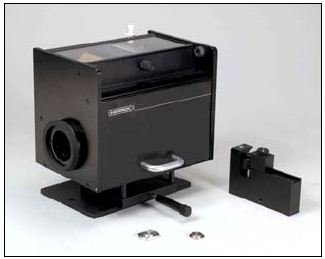Figure 3. The Seagull™ Variable Angle Reflectance Accessory.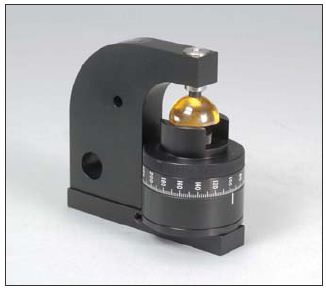Figure 4. The ATR Rotator for the Seagull.

The Seagull pressure applicator was manually tightened and subsequently used to compress the sample against the ATR crystal. The ATR Rotator is developed in such a way that the ATR hemisphere oscillates with the sample, without affecting the contact between the sample and the ATR hemisphere as the sample rotates around the z axis.

The ATR Rotator includes a scale in 1° increments to represent the sample rotation and it can be turned a full 360°. For all orientations of the sample, s-polarized light kept at a fixed angle of incidence was utilized. By this way, the strength and the direction of the electric field remain similar for all the measurements and can be compared directly.

In simper terms, the ratio of absorbances for the two different angles is observed to be the same as the ratio of the fractions of bonds oriented along these directions. During the course of the experiment, if the angle of incidence changed, the sample’s electric fields would modify according to (1) and (2) equations and corrections would have to be made to the quantified absorbances to compare them directly. An FT-IR spectrometer integrated with a DTGS detector was used to record all the spectra. The spectrometer was able to collect 32 scans at an 8cm-1 resolution.

## Results and Discussion

Figure 5 shows the two spectra at the extremes of spectral variations. An interesting aspect of these spectra is the collective variation in the strength of absorbance for the two orientations.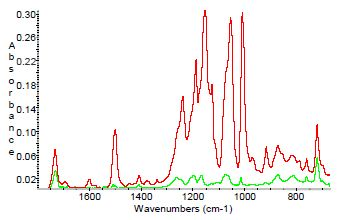Figure 5. Spectra recorded at a 160° (green) and a 260° (red) rotation of the sample around the z-axis, showing the extremes of the spectral variation.

It looks as if all the important bonds in the sample are oriented along the direction, as defined by the electric field at the sample rotation marked as 260°. A number of peaks seems to have equal strength within a factor of two; however, a large number of peaks shows significant dependence on the sample orientation.

Bonds that are strongly oriented are oriented along the same direction. Figure 6 shows the difference of peak height with angle, illustrating that the peak at 1500cm-1 is changing with respect to the angle of orientation. Figure 7 displays all 37 spectra of the sample, obtained in 100 increments, and hence, represent a complete 360° sample rotation.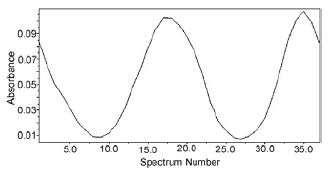Figure 6. Variation in absorbance of the peak at 1500 cm-1 as a function of sample rotation around the z axis. The spectra were taken in 10° steps so the angular range is 360°.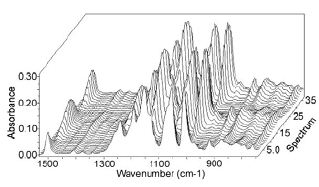Figure 7. Spectra of oriented sample in 10° steps over the 360° angle.

This makes it possible to view which bonds have orientation and which bonds do not. The bond at 1000cm-1 has a high degree of orientation, whereas the bond at 760cm-1 seems to be unaffected by the orientation. The absorbance is anticipated to be a function proportional to cos 2 (Ψ-β), where angle β denotes orientation of the projection of the bond into the xy plane relating to the starting orientation, and the angle Ψ determines the sample rotation from a random starting orientation.

In case another bond exhibited varied orientation of its projection into the xy plane, the same plot for that specific bond would be phase shifted with regard to the 1500cm-1 bond, and the angle of shift would indicate the angle between the projections of the two bonds into the xy plane.

The orientation of a molecular bond is not usually perfect. In other words, all the dipoles are not aligned in the same direction. Most dipoles may point in a specific direction, whilst the rest of the dipoles may point in arbitrary directions. The dipoles may also have the same orientation, but each would be slightly out of the alignment.

In such a case, the plot illustrated in Figure 5 would have minima in proportion to the fraction of nonaligned dipole components. The bond has strength difference from 0.10A in the aligned direction to 0.01A in the perpendicular direction, and is highly oriented with an orientation fraction f = 0.75. This indicates that three quarters of the dipoles related to this bond are perfectly oriented.

## Conclusion

This article has shown how ATR, utilizing the Harrick Seagull pressure applicator with its ATR Rotator and wire grid polarizer, allows comprehensive analysis of oriented samples, thus simplifying analyses of bond orientations referenced to the external sample dimensions.This information has been sourced, reviewed and adapted from materials provided by Harrick Scientific Products, Inc.

## Citations

• APA

Harrick Scientific Products, Inc.. (2019, December 13). Using an ATR Rotator to Study Oriented Samples. AZoM. Retrieved on January 25, 2020 from https://www.azom.com/article.aspx?ArticleID=12273.

• MLA

Harrick Scientific Products, Inc.. "Using an ATR Rotator to Study Oriented Samples". AZoM. 25 January 2020. <https://www.azom.com/article.aspx?ArticleID=12273>.

• Chicago

Harrick Scientific Products, Inc.. "Using an ATR Rotator to Study Oriented Samples". AZoM. https://www.azom.com/article.aspx?ArticleID=12273. (accessed January 25, 2020).

• Harvard

Harrick Scientific Products, Inc.. 2019. Using an ATR Rotator to Study Oriented Samples. AZoM, viewed 25 January 2020, https://www.azom.com/article.aspx?ArticleID=12273.